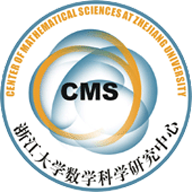(12017)A Critical Exponent of Fujita Type for a Nonlinear Reaction-Di usion System on Riemannian Manifold

Abstract
In this paper, we study the global existence and nonexistence of positive solutions to the following nonlinear reaction-di usion system
8>>>>>>>>>>>><
>>>>>>>>>>>>:
ut 􀀀 u = W(x)vp + S(x) in Mn  (0;1);
vt 􀀀 v = F(x)ud + G(x) in Mn  (0;1);
u(x; 0) = u0(x) in Mn;
v(x; 0) = v0(x) in Mn;
where Mn (n  3) is a non-compact complete Riemannian manifold,  is the Laplace-Beltrami operator, and S(x);G(x) are non-negative L1 loc functions. We assume that both u0(x) and v0(x) are non-negative , smooth and bounded functions, constants p ; d > 1. When p = d, there is an exponent p which is critical in the following sense. when p 2 (1; p], the above problem has no global positive solution for any non-negative constants S(x);G(x) not identically zero; when p 2 [p;1), the problem has a global positive solution for some S(x);G(x) > 0 and
u0(x); v0(x)  0.
Key words and phrases: critical exponent; reaction-di usion system; Riemannian manifold.

• A Critical Exponent of Fujita Type for a Nonlinear Reaction-Diffusion System on Riemannian Manifold .pdf